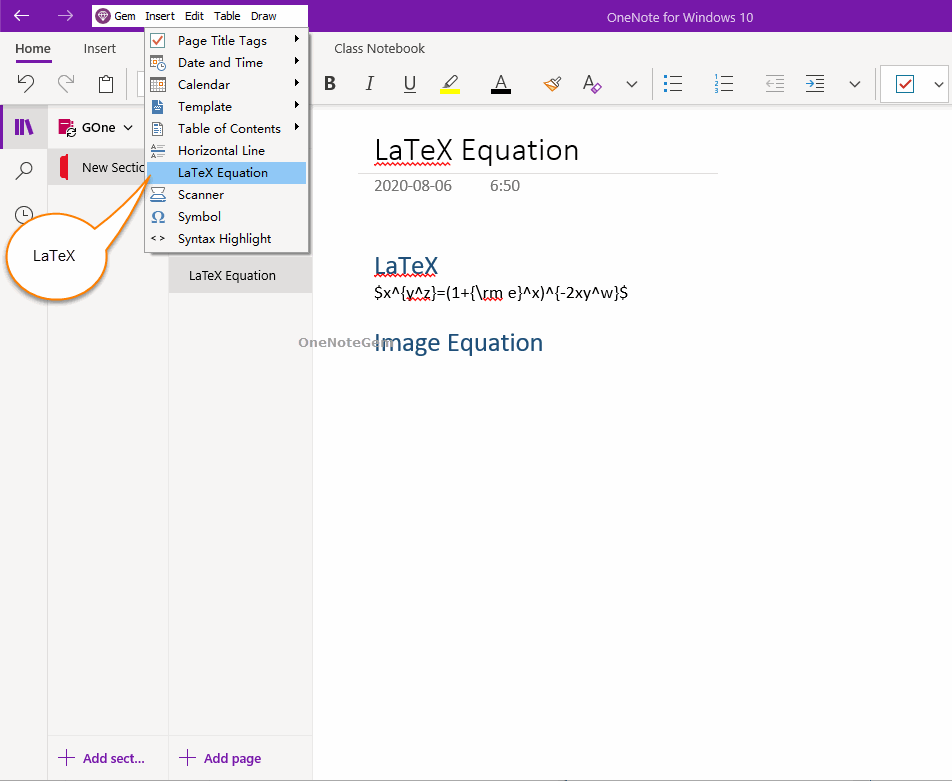### UWP: Copy LaTeX to Generate Image Equation into OneNote

There are many LaTeX expressions in the web page, and they are not easy to generate to equation quickly in OneNote.
Gem Menu for OneNote UWP add-in now offers a "LaTeX Equation" feature that allows you to copy LaTeX expressions from web pages, convert them into image equations and put them in OneNote.

## LaTeX Equation of Gem Menu

The LaTeX Equation of the Gem Menu located in the "Insert" menu -> "LaTeX Equation" menu item.## LaTeX Expression Generate Image Equation

#### LaTeX Input Box

You can paste the LaTeX expression from the web page.
Expression requirements: Enclose LaTeX by \$...\$ or \$\$...\$\$ or (…) or […].
For example:

\$x^{y^z}=(1+{ m e}^x)^{-2xy^w}\$

#### Size:

Zoom in or out the equation as a percentage.## Demonstration